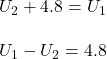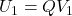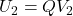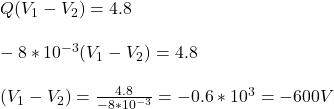A charged particle (q = 8.0 mC), which moves in a region where the only force acting on the particle is an electric force, is released from

Question

A charged particle (q = 8.0 mC), which moves in a region where the only force acting on the particle is an electric force, is released from rest at point A. At point B the kinetic energy of the particle is equal to 4.8 J. What is the electric potential difference VB  VA?

in progress 0
6 months 2021-07-31T17:00:35+00:00 1 Answers 42 views 0

1. The potential difference will be 600 V.

Explanation:

Here the charged particle is said to exhibit a charge of -8 mC. So when it moves from point A to point B, it gains a kinetic energy of 4.8 J. Also, the charged particle is said to exhibit an electrostatic potential energy at points A and B.

The particle will obey conservation of energy. So the sum of energy exhibited by the particle at point B will be equal to the sum of energy exhibited by the particle at point A.

At point A, the kinetic energy is zero and the electrostatic energy will be represented as U₁.

Then, the total energy at point A = U₁

Similarly, at point B, the charged particle will exhibit kinetic energy as well as electrostatic potential energy (U₂).

Total energy exhibited by the charged particles at point B = U₂ + K.E = U₂+4.8.

Then, as per law of conservation of energy:It is known that electrostatic potential energy is the product of charge with the potential at that point.

So,andThen,Then, V₂-V₁ will be 600 V.

Thus, the potential difference will be 600 V.